# Pair Trading Strategy And Backtesting Using Quantstrat [EPAT PROJECT]

This article is the final project submitted by the author as a part of his coursework in Executive Programme in Algorithmic Trading (EPAT) at QuantInsti. Do check our Projects page and have a look at what our students are building.

### A Recent Webinar Presentation by Marco Nicolas Dibo

This insightful webinar on pairs trading and sourcing data covers the basics of pair trading strategy followed by two examples. In the first example, Marco covers the pairs trading strategy for different stocks traded on the same exchange, and in the second example, Marco has illustrated the pairs strategy for different commodity futures traded on different exchanges. Marco also details the different data sources including Quandl which can be used for creating trading strategies.

### AuthorMarco has spent his career as a trader and portfolio manager, with a particular focus in equity and derivatives markets. He specializes in quantitative finance and algorithmic trading and currently serves as head of the Quantitative Trading Desk and Vice-president of Argentina Valores S.A. Marco is also Co-Founder and CEO of Quanticko Trading SA, a firm devoted to the development of high frequency trading strategies and trading software. Marco holds a BS in Economics and an MSc in Finance from the University of San Andrés.

### Introduction

One of my favorite classes during EPAT was the one on statistical arbitrage, so the pair trading strategy seemed a nice idea for me. My strategy triggers new orders when the pair ratio of the prices of the stocks diverge from the mean. But in order to work, we first have to test for the pair to be cointegrated. If the pair ratio is cointegrated, the ratio is mean-reverting and the greater the dispersion from its mean, the higher the probability of a reversal, which makes the trade more attractive. I chose the following pair of stocks:

• Bank of America (BAC)
• Citigroup (C)

The idea is the following: If we find two stocks that are correlated (they correspond to the same sector), and the pair ratio diverges from a certain threshold, we short the stock that is expensive and buy the one that is cheap. Once they converge to the mean, we close the positions and profit from the reversal.

The logic is simple. The algorithm calculates the daily Z-score for every pair of stocks. The Z-score is the number of standard deviations that the pair ratio has diverged from its mean:

Z = (R - μ) / σ

Where R is the  price ratio of both stocks, μ is the mean of the ratio and σ is the standard deviation of the price ratio.

Once the Z-score is outside of a certain threshold, we fulfill the first condition required for sending an order.

But the algorithm must also meet a second condition: It calculates the rolling Augmented Dickey Fuller test for the pair of stocks. More specifically, it gets the p-value from the test. Then it compares it with a defined significance level (alpha) and if the p-value is less than the alpha, it means that the price ratio series are stationary and the second condition is met. If both conditions are met, then the algorithm buys the loser and sells the winner. The exit rules apply at a certain Z-score threshold. For the optimization of the strategy the variables that I used were the following:

1. Z-Score entry thresholds
2. Z-Score exit thresholds
3. Second condition (cointegration) True or False

### Code details and In-Sample Backtest:

The in-sample period for backtesting was 01-01-2009 till 31-12-2012. The Z-score was calculated using the following parameters:

1. Moving average of the price ratio: 20 days
2. Standard Deviation of the price ratio: 20 days
3. ADF test window: 60 days
4. Initial Equity = 100.000 USD

When we short the spread we are selling "C" and buying "BAC" and when we buy the spread we are doing the opposite. I used quantstrat library  for backtesting the strategy. Let us dive into the code:

# Load libraries

library(quantstrat)
library(tseries)
library(PerformanceAnalytics)# .blotter holds the portfolio and account object and .strategy holds the orderbook and strategy object

.blotter <- new.env()
.strategy <- new.env()# fetch market data and plot the spread

symb1 <- 'C'
symb2 <- 'BAC'

stock(symbols, currency = 'USD', multiplier = 1)

legend(x=5, y=50, legend=c("EMA 20"),
fill=c("blue"), bty="n")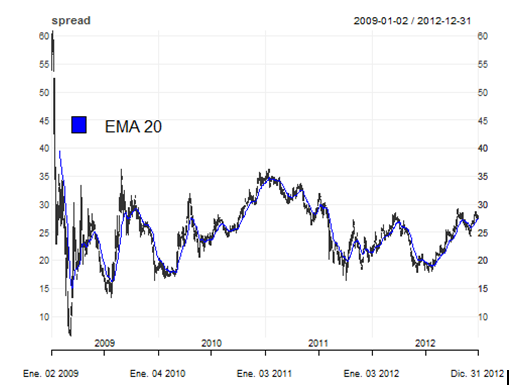As mentioned earlier, I will use the quantstrat library for the optimization of my strategy. In order to use quantstrat we first have to define and initialize instruments, strategy, portfolio, account and orders:

#Inititalize strategy, portfolio, account and orders

qs.strategy <- 'pairStrat'
initPortf(qs.strategy, symbols = symbols, initDate=initDate)

initAcct(qs.strategy, portfolios=qs.strategy, initDate=initDate,initEq=initEq)

initOrders(qs.strategy,initDate=initDate)
# Save strategy
strategy(qs.strategy, store = TRUE)
# rm.strat(pairStrat) # only when trying a new test
ls(.blotter) # .blotter holds the portfolio and account object

ls(.strategy) # .strategy holds the orderbook and strategy object

Then we calculate and add to the strategy our two indicators to the strategy:

• Z-Score

• ADF Test (True or False)

# a) Z-Score
PairRatio <- function(x) { #returns the ratio of close prices for 2 symbols
x1 <- get(x)
x2 <- get(x)
rat <- log10(Cl(x1) / Cl(x2))
colnames(rat) <- 'Price.Ratio'
rat
}

Price.Ratio <- PairRatio(c(symb1,symb2))

MaRatio <- function(x){

Mavg <- rollapply(x, N , mean)
colnames(Mavg) <- 'Price.Ratio.MA'
Mavg
}

Price.Ratio.MA <- MaRatio(Price.Ratio)

Sd <- function(x){

Stand.dev <- rollapply(x, N, sd)
colnames(Stand.dev) <- "Price.Ratio.SD"
Stand.dev
}

Price.Ratio.SD <- Sd(Price.Ratio)

ZScore <- function(x){

a1 <- x$Price.Ratio b1 <- x$Price.Ratio.MA
c1 <- x$Price.Ratio.SD z <- (a1-b1)/c1 colnames(z)<- 'Z.Score' z }# b) Augmented Dickey Fuller ft2<-function(x){ adf.test(x)$p.value
}

Pval <- function(x){

Augmented.df <- rollapply(x, width = N.ADF, ft2)
colnames(Augmented.df) <- "P.Value"
Augmented.df
}

P.Value <- Pval(Price.Ratio)

add.indicator(strategy = qs.strategy, name = "ZScore", arguments =
list(x=merge(Price.Ratio,Price.Ratio.MA,Price.Ratio.SD)))

add.indicator(strategy = qs.strategy, name = "Pval", arguments =
list(x=quote(Price.Ratio)))



In the following chart we can see the evolution of the Z-score during the period and the possible values for the threshold where the ratio reverts to the mean and the extreme values. I set some lines in the +/-2 Z-score threshold, where it seems to be a reversal of the pair ratio. This value of the z-score means that the pair ratio is +/- standard deviations from its mean.

Z.Score <- ZScore(x=merge(Price.Ratio,Price.Ratio.MA,Price.Ratio.SD))
plot(main = "Z-Score Time Series", xlab = "Date" , ylab = "Z-Score",Z.Score, type = "l" )
abline(h = 2, col = 2, lwd = 3 ,lty = 2)
abline(h = -2, col = 3, lwd = 3 ,lty = 2)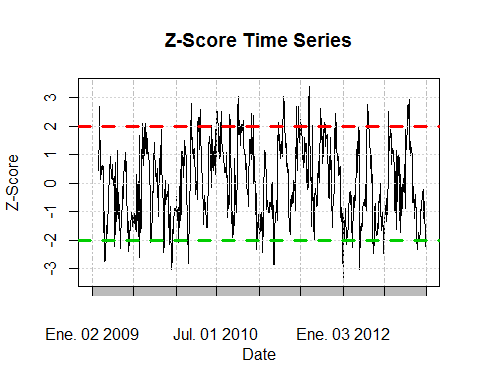Now we set our optimization variables:

alpha = 1 # We set it to 0.1 if we want a 10% significance level. If we want to set the ADF test (second condition)
#off, we just change it to "1", in that case the p-value will always be lower than the significance level and the # and the strategy will not require the pair to be cointegrated.# Z-Score entry and exit thresholds:

exitlong = 1
exitshort = 1

Before running our backtest, we have to add the signals, position limits and rules of our strategy:

relationship="lt", cross=FALSE),label="longEntryZ")

relationship="lt", cross=FALSE),label="PEntry")

arguments=list(columns=c("longEntryZ", "PEntry"), cross=FALSE),
label="longEntry")

relationship="gt", cross=FALSE),label="longExit")

relationship="gt", cross=FALSE),label="shortEntryZ")

label="shortEntry")

relationship="lt", cross=FALSE),label="shortExit")

timestamp = initDate,
maxpos = 3000,
longlevels = 1,
minpos = -3000)

sigval=TRUE, orderqty=3000,  osFUN = osMaxPos, replace = FALSE, ordertype='market',
orderside='long', prefer = "open"), type='enter' )

sigval=TRUE, orderqty=-3000,  osFUN = osMaxPos, replace = FALSE,ordertype='market',
orderside='short', prefer = "open"), type='enter')

sigval=TRUE, orderqty= 'all', ordertype='market', orderside='short', prefer = "open"), type='exit')

sigval=TRUE, orderqty= 'all' , ordertype='market', orderside='long', prefer = "open"), type='exit')

summary(get.strategy(qs.strategy))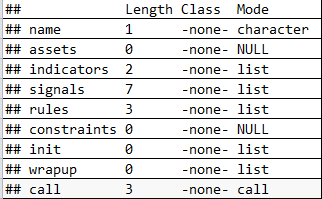As we can see from our summary there are 2 indicators, 7 signals and 3 rules defined in our strategy. Now we can run the backtest, check the transactions and the performance of our strategy.

applyStrategy(strategy = qs.strategy, portfolios = qs.strategy, mktdata = spread)

tns <-getTxns(Portfolio=qs.strategy, Symbol= symbols)#Update portfolio, account, equity
updatePortf(qs.strategy)

updateAcct(qs.strategy)

updateEndEq(qs.strategy)



The optimization was done with the following values for the variables: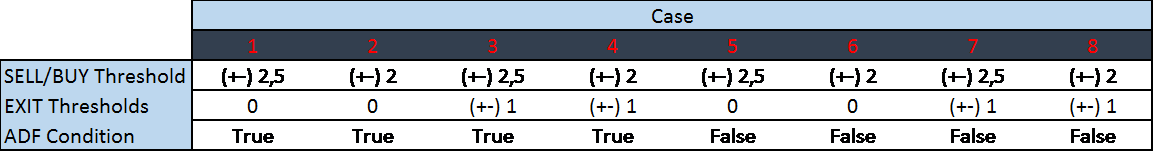From the in-sample backtest we got the following results: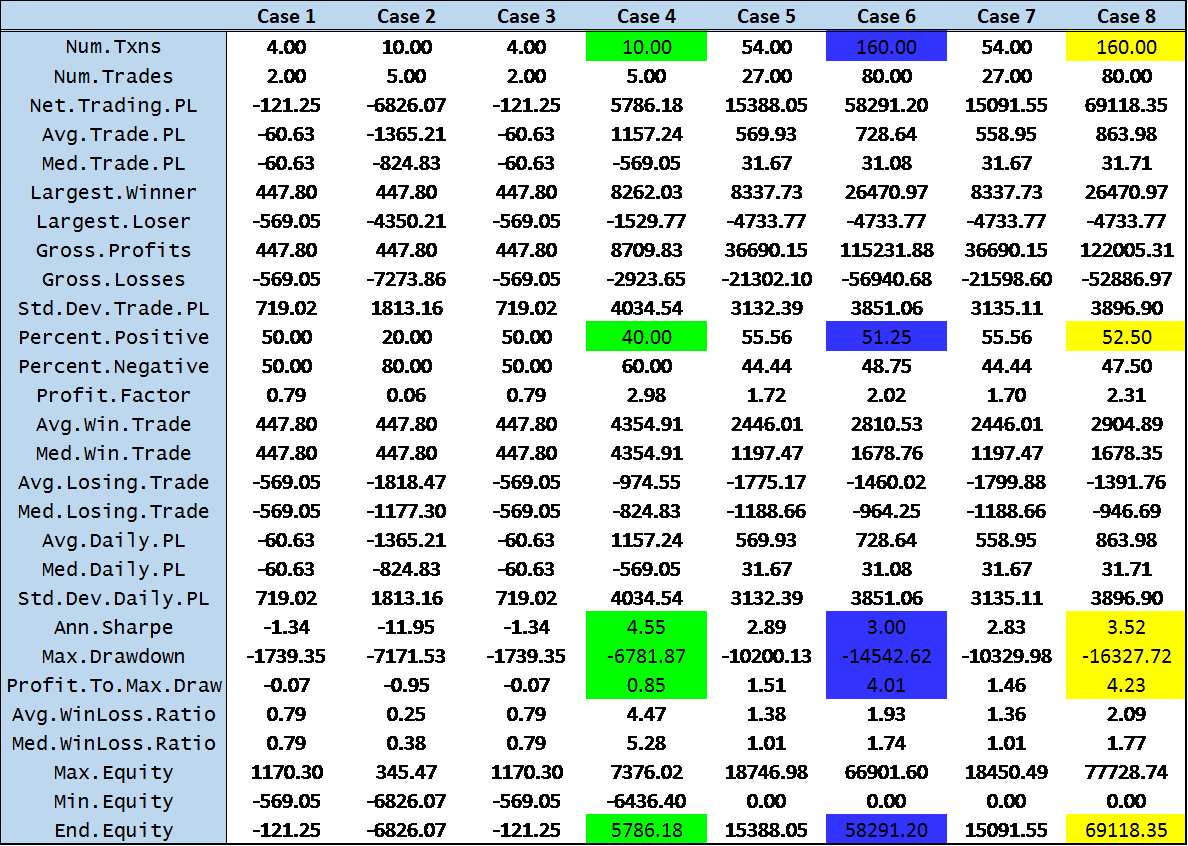From this table we can get the values for the variables that optimize the strategy. At first sight it seems that there are 3 candidates (case 4, case 6 and case 8). If we compare between cases 6 and 8 we arrive to the conclusion that case 8 is the best one as it has a greater annualized Sharpe ratio and profit to max drawdown, a higher percentage of positive trades, a greater end equity and with the same number of trades. So now we are left with only 2 candidates: 4 and 8. If we would only be checking for the one with the greatest annualized Sharpe ratio, we would prefer case 4. Case 8 also doesn't take into account that the series must be cointegrated, and case 4 does, so this would be another plus for case 4. But if we take into account the number of transactions, the profit to max drawdown, the end equity, the percentage of positive trades and the fact that the difference in the Sharpe ratio is not a big difference we would definitely select case 8 as our best candidate.

### Out of Sample Backtest:

Now that we have optimized the strategy and obtained the optimal values for the parameters, we can run an out of sample blacktest and see how the strategy performs. The out of sample period for the back test goes from the 01-01-2013 to the 31-12-2015 and the optimized values for the thresholds and rules were the following:

• Z-Score Buy Threshold = -2
• Z-Score Sell Threshold = 2
• Z-Score Long Exit Threshold = -1
• Z-Score Short Exit Threshold = 1

The following chart show us the different transactions, the end equity and the drawdown results for our strategy:

chart.P2 = function (Portfolio, Symbol, Dates = NULL, ..., TA = NULL)
{
pname <- Portfolio
Portfolio <- getPortfolio(pname)
if (missing(Symbol))
Symbol <- ls(Portfolio$symbols)[] else Symbol <- Symbol Prices = get(Symbol) if (!is.OHLC(Prices)) { if (hasArg(prefer)) prefer = eval(match.call(expand.dots = TRUE)$prefer)
else prefer = NULL
Prices = getPrice(Prices, prefer = prefer)
}
freq = periodicity(Prices)
switch(freq$scale, seconds = { mult = 1 }, minute = { mult = 60 }, hourly = { mult = 3600 }, daily = { mult = 86400 }, { mult = 86400 }) if (!isTRUE(freq$frequency * mult == round(freq$frequency, 0) * mult)) { n = round((freq$frequency/mult), 0) * mult
}
else {
n = mult
}
tzero = xts(0, order.by = index(Prices[1, ]))
if (is.null(Dates))
Dates <- paste(first(index(Prices)), last(index(Prices)),
sep = "::")
Portfolio$symbols[[Symbol]]$txn <- Portfolio$symbols[[Symbol]]$txn[Dates]
Portfolio$symbols[[Symbol]]$posPL <- Portfolio$symbols[[Symbol]]$posPL[Dates]
Trades = Portfolio$symbols[[Symbol]]$txn$Txn.Qty Buys = Portfolio$symbols[[Symbol]]$txn$Txn.Price[which(Trades >
0)]
Sells = Portfolio$symbols[[Symbol]]$txn$Txn.Price[which(Trades < 0)] Position = Portfolio$symbols[[Symbol]]$txn$Pos.Qty
if (nrow(Position) < 1)
stop("no transactions/positions to chart")
if (as.POSIXct(first(index(Prices))) < as.POSIXct(first(index(Position))))
Position <- rbind(xts(0, order.by = first(index(Prices) -
1)), Position)
Positionfill = na.locf(merge(Position, index(Prices)))
CumPL = cumsum(Portfolio$symbols[[Symbol]]$posPL\$Net.Trading.PL)
if (length(CumPL) > 1)
CumPL = na.omit(na.locf(merge(CumPL, index(Prices))))
else CumPL = NULL
if (!is.null(CumPL)) {
CumMax <- cummax(CumPL)
Drawdown <- -(CumMax - CumPL)
Drawdown <- rbind(xts(-max(CumPL), order.by = first(index(Drawdown) -
1)), Drawdown)
}
else {
Drawdown <- NULL
}
if (!is.null(Dates))
Prices = Prices[Dates]
chart_Series(Prices, name = Symbol, TA = TA, ...)
(add_TA(Buys, pch = 2, type = "p", col = "green", on = 1))
if (!is.null(nrow(Sells)) && nrow(Sells) >= 1)
(add_TA(Sells, pch = 6, type = "p", col = "red", on = 1))
if (nrow(Position) >= 1) {
(add_TA(Positionfill, type = "h", col = "blue", lwd = 2))
(add_TA(Position, type = "p", col = "orange", lwd = 2,
on = 2))
}
if (!is.null(CumPL))
(add_TA(CumPL, col = "darkgreen", lwd = 2))
if (!is.null(Drawdown))
(add_TA(Drawdown, col = "darkred", lwd = 2, yaxis = c(0,
-max(CumMax))))
plot(current.chob())
}

chart.P2(qs.strategy, "spread", prefer = "close")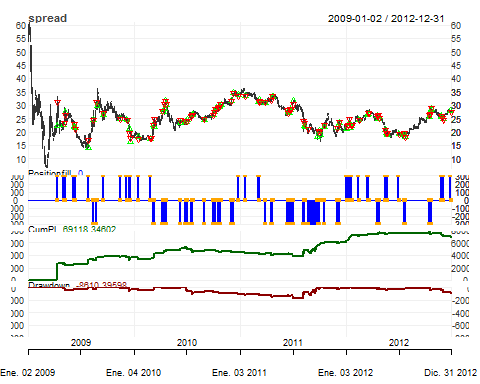From the table below we can see that the results from the out of sample backtest are not as good as the ones we got from the in sample backtest.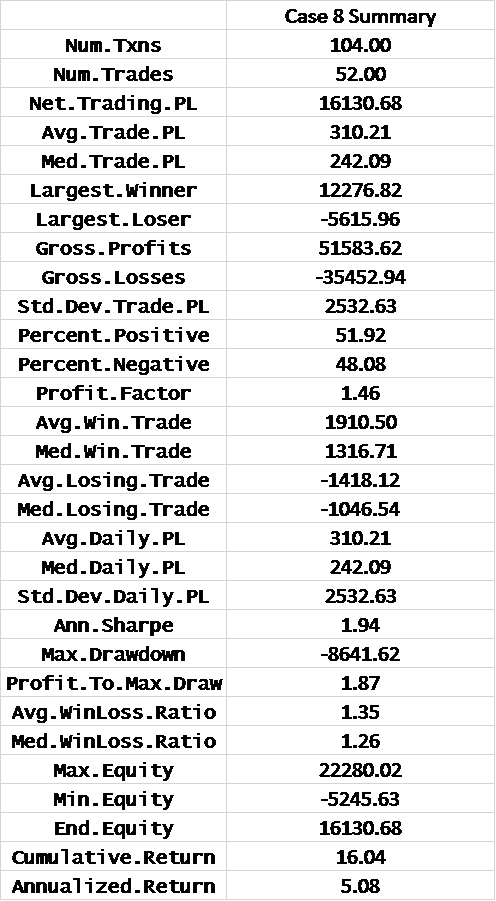The annualized Sharpe ratio is still positive but smaller than the 3.52 that we got before. The profit to max drawdown is quite worse than the 4.23 but the max drawdown decreased from 16327 to 8641. Our strategy delivers a cumulative return of 16.04% and annualized return of 5.08% during the three years that it was deployed.

returns <- PortfReturns(qs.strategy)
charts.PerformanceSummary(returns, geometric=FALSE, wealth.index=TRUE, main = "Pair Strategy Returns")

### Conclusion

The idea when I started the Executive Program in Algorithmic trading was to learn how to model a quantitative trading strategy, backtest it and then optimize it. Thanks to my professors and QuantInsti staff I feel that the objective was accomplished. Everything in the course was excellent and would recommend it to everyone interested in learning algorithmic trading.

Let us take you through a story of an MBA in finance student that will inspire you towards a successful Algorithmic trading career with the help of EPAT programme. This success story covers the journey of Jayalaxmi who was a Financial Analyst prior to enrolling for the EPAT programme. After successful completion of the programme, Jayalaxmi joined WorldQuant LLC which is headquartered in Old Greenwich, Connecticut.